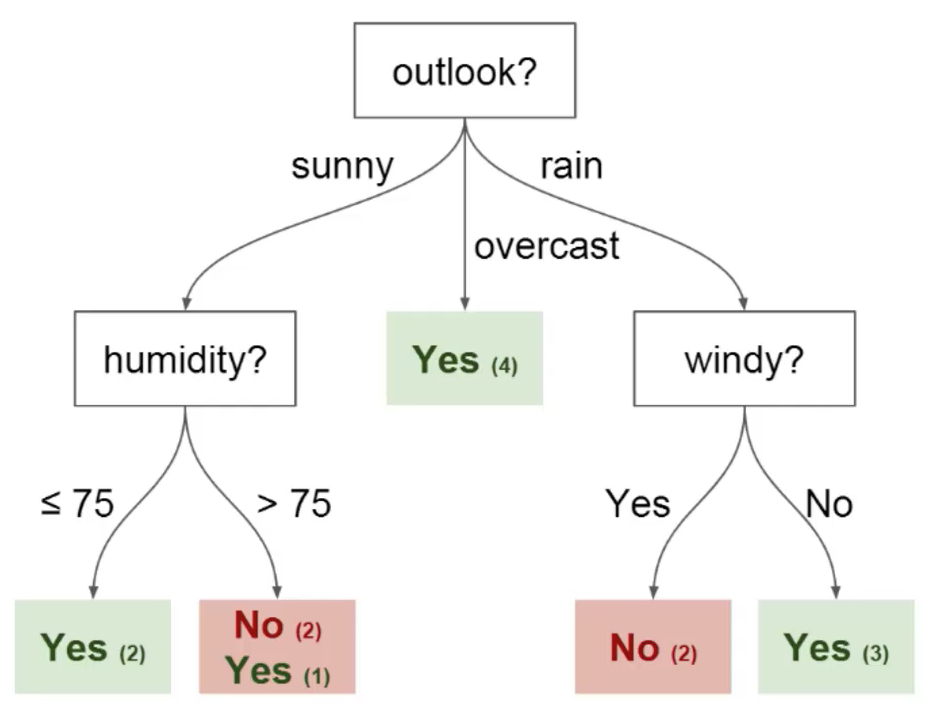# Introduction to Decision Trees and Random Forests

Hey - Nick here! This page is a free excerpt from my new eBook Pragmatic Machine Learning, which teaches you real-world machine learning techniques by guiding you through 9 projects.

This tutorial will serve as a theoretical introduction to decision trees and random forests.

In the next section of this course, you will build your first decision tree machine learning model in Python.

## What Are Tree Methods?

Before we dig into the theoretical underpinnings of tree methods in machine learning, it is helpful to start with an example.

Imagine that you play basketball every Monday. Moreover, you always invite the same friend to come play with you.

Sometimes the friend actually comes. Sometimes he doesn't.

The decision on whether or not to come depends on numerous factors, like weather, temperature, wind, and fatigue. You start to notice these features and begin tracking them beside his decision to play or not.

You can use this data to predict whether or not your friend will show up to play basketball. One technique you could use is a decision tree. Here's what this decision tree would look like:Every decision tree has two types of elements:

• `Nodes`: locations where the tree splits according to the value of some attribute
• `Edges`: the outcome of a split to the next node

You can see in the image above that there are nodes for `outlook`, `humidity` and `windy`. There is an edge for each potential value of each of those attributes.

Here are two other pieces of decision tree terminology that you should understand before proceeding:

• `Root`: the node that performs the first split
• `Leaves`: terminal nodes that predict the final outcome

You now have a basic understanding of what decision trees are. We will learn about how to build decision trees from scratch in the next section.

## How to Build Decision Trees From Scratch

Building decision trees is harder than you might imagine. This is because deciding which features to split your data on (which is a topic that belongs to the fields of Entropy and Information Gain) is a mathematically complex problem.

To address this, machine learning practitioners typically use many decision trees using a random sample of features chosen as the split.

Said differently, a new random sample of features is chosen for every single tree at every single split. This technique is called `random forests`.

In general, practitioners typically chose the size of the random sample of features (denoted `m`) to be the square root of the number of total features in the data set (denoted `p`). To be succinct, `m` is the square root of `p`, and then a specific feature is randomly selected from `m`.

If this does not make complete sense right now, do not worry. It will be more clear when we build our first random forest in the next tutorial of this course.

## The Benefits of Using Random Forests

Imagine that you're working with a data set that has one very strong feature. Said differently, the data set has one feature that is much more predictive of the final outcome than the other features in the data set.

If you're building your decision trees manually, then it makes sense to use this feature as the top split of the decision tree. This means that you'll have multiple trees whose predictions are highly correlated.

We want to avoid this since taking the average of highly correlated variables does not significantly reduce variance. By randomly selecting features for each tree in a random forest, the trees become decorrelated and the variance of the resulting model is reduced.

## Final Thoughts

In this tutorial, you had your first exposure to tree methods in machine learning.

Here is a brief summary of what we discussed in this article:

• An example of a problem that you could predict using decision trees
• The elements of a decision tree: `nodes`, `edges`, `roots`, and `leaves`
• How taking random samples of decision tree features allows us to build a random forest
• Why using random forests to decorrelate variables can be helpful for reducing the variance of your final model